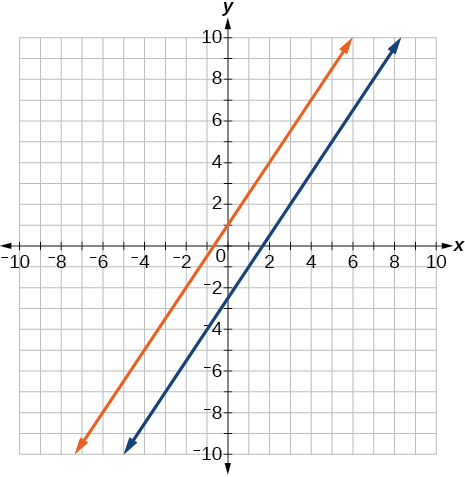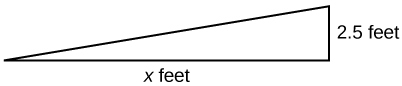# 2.2 Linear equations in one variable  (Page 8/15)

 Page 8 / 15

$\frac{x+2}{4}-\frac{x-1}{3}=2$

$x=-14$

For the following exercises, solve each rational equation for $\text{\hspace{0.17em}}x.\text{\hspace{0.17em}}$ State all x -values that are excluded from the solution set.

$\frac{3}{x}-\frac{1}{3}=\frac{1}{6}$

$2-\frac{3}{x+4}=\frac{x+2}{x+4}$

$x\ne -4;$ $x=-3$

$\frac{3}{x-2}=\frac{1}{x-1}+\frac{7}{\left(x-1\right)\left(x-2\right)}$

$\frac{3x}{x-1}+2=\frac{3}{x-1}$

$x\ne 1;$ when we solve this we get $\text{\hspace{0.17em}}x=1,$ which is excluded, therefore NO solution

$\frac{5}{x+1}+\frac{1}{x-3}=\frac{-6}{{x}^{2}-2x-3}$

$\frac{1}{x}=\frac{1}{5}+\frac{3}{2x}$

$x\ne 0;$ $x=\frac{-5}{2}$

For the following exercises, find the equation of the line using the point-slope formula.

Write all the final equations using the slope-intercept form.

$\left(0,3\right)\text{\hspace{0.17em}}$ with a slope of $\text{\hspace{0.17em}}\frac{2}{3}$

$\left(1,2\right)\text{\hspace{0.17em}}$ with a slope of $\text{\hspace{0.17em}}\frac{-4}{5}$

$y=\frac{-4}{5}x+\frac{14}{5}$

x -intercept is 1, and $\text{\hspace{0.17em}}\left(-2,6\right)$

y -intercept is 2, and $\text{\hspace{0.17em}}\left(4,-1\right)$

$y=\frac{-3}{4}x+2$

$\left(-3,10\right)\text{\hspace{0.17em}}$ and $\text{\hspace{0.17em}}\left(5,-6\right)$

$y=\frac{1}{2}x+\frac{5}{2}$

parallel to $\text{\hspace{0.17em}}y=2x+5\text{\hspace{0.17em}}$ and passes through the point $\text{\hspace{0.17em}}\left(4,3\right)$

perpendicular to $\text{\hspace{0.17em}}\text{3}y=x-4\text{\hspace{0.17em}}$ and passes through the point $\text{\hspace{0.17em}}\left(-2,1\right)$ .

$y=-3x-5$

For the following exercises, find the equation of the line using the given information.

$\left(-2,0\right)\text{\hspace{0.17em}}$ and $\text{\hspace{0.17em}}\left(-2,5\right)$

$\left(1,7\right)\text{\hspace{0.17em}}$ and $\text{\hspace{0.17em}}\left(3,7\right)$

$y=7$

The slope is undefined and it passes through the point $\text{\hspace{0.17em}}\left(2,3\right).$

The slope equals zero and it passes through the point $\text{\hspace{0.17em}}\left(1,-4\right).$

$y=-4$

The slope is $\text{\hspace{0.17em}}\frac{3}{4}\text{\hspace{0.17em}}$ and it passes through the point $\text{\hspace{0.17em}}\text{(1,4)}\text{.}$

$\left(-1,3\right)\text{\hspace{0.17em}}$ and $\text{\hspace{0.17em}}\left(4,-5\right)$

$8x+5y=7$

## Graphical

For the following exercises, graph the pair of equations on the same axes, and state whether they are parallel, perpendicular, or neither.

$\begin{array}{l}\\ \begin{array}{l}y=2x+7\hfill \\ y=\frac{-1}{2}x-4\hfill \end{array}\end{array}$

$\begin{array}{l}3x-2y=5\hfill \\ 6y-9x=6\hfill \end{array}$Parallel

$\begin{array}{l}y=\frac{3x+1}{4}\hfill \\ y=3x+2\hfill \end{array}$

$\begin{array}{l}x=4\\ y=-3\end{array}$Perpendicular

## Numeric

For the following exercises, find the slope of the line that passes through the given points.

$\left(5,4\right)\text{\hspace{0.17em}}$ and $\text{\hspace{0.17em}}\left(7,9\right)$

$\left(-3,2\right)\text{\hspace{0.17em}}$ and $\text{\hspace{0.17em}}\left(4,-7\right)$

$m=\frac{-9}{7}$

$\left(-5,4\right)\text{\hspace{0.17em}}$ and $\text{\hspace{0.17em}}\left(2,4\right)$

$\left(-1,-2\right)\text{\hspace{0.17em}}$ and $\text{\hspace{0.17em}}\left(3,4\right)$

$m=\frac{3}{2}$

$\text{\hspace{0.17em}}\left(3,-2\right)$ and $\text{\hspace{0.17em}}\left(3,-2\right)$

For the following exercises, find the slope of the lines that pass through each pair of points and determine whether the lines are parallel or perpendicular.

${m}_{1}=\frac{-1}{3},\text{ }{m}_{2}=3;\text{ }\text{Perpendicular}\text{.}$

## Technology

For the following exercises, express the equations in slope intercept form (rounding each number to the thousandths place). Enter this into a graphing calculator as Y1, then adjust the ymin and ymax values for your window to include where the y -intercept occurs. State your ymin and ymax values.

$0.537x-2.19y=100$

$y=0.245x-45.662.\text{\hspace{0.17em}}$ Answers may vary.

$4,500x-200y=9,528$

$\frac{200-30y}{x}=70$

$y=-2.333x+6.667.\text{\hspace{0.17em}}$ Answers may vary.

## Extensions

Starting with the point-slope formula $\text{\hspace{0.17em}}y-{y}_{1}=m\left(x-{x}_{1}\right),$ solve this expression for $\text{\hspace{0.17em}}x\text{\hspace{0.17em}}$ in terms of $\text{\hspace{0.17em}}{x}_{1},y,{y}_{1},$ and $\text{\hspace{0.17em}}m.$

Starting with the standard form of an equation solve this expression for y in terms of $\text{\hspace{0.17em}}A,B,C,\text{\hspace{0.17em}}$ and $\text{\hspace{0.17em}}x.\text{\hspace{0.17em}}$ Then put the expression in slope-intercept form.

$y=\frac{-A}{B}x+\frac{C}{B}$

Use the above derived formula to put the following standard equation in slope intercept form: $\text{\hspace{0.17em}}7x-5y=25.$

Given that the following coordinates are the vertices of a rectangle, prove that this truly is a rectangle by showing the slopes of the sides that meet are perpendicular.

$\left(-1,1\right),\left(2,0\right),\left(3,3\right)\text{,}$ and $\text{\hspace{0.17em}}\left(0,4\right)$

Yes they are perpendicular.

Find the slopes of the diagonals in the previous exercise. Are they perpendicular?

## Real-world applications

The slope for a wheelchair ramp for a home has to be $\text{\hspace{0.17em}}\frac{1}{12}.\text{\hspace{0.17em}}$ If the vertical distance from the ground to the door bottom is 2.5 ft, find the distance the ramp has to extend from the home in order to comply with the needed slope.30 ft

If the profit equation for a small business selling $\text{\hspace{0.17em}}x\text{\hspace{0.17em}}$ number of item one and $\text{\hspace{0.17em}}y\text{\hspace{0.17em}}$ number of item two is $\text{\hspace{0.17em}}p=3x+4y,$ find the $\text{\hspace{0.17em}}y\text{\hspace{0.17em}}$ value when

For the following exercises, use this scenario: The cost of renting a car is $45/wk plus$0.25/mi traveled during that week. An equation to represent the cost would be $\text{\hspace{0.17em}}y=45+.25x,$ where $\text{\hspace{0.17em}}x\text{\hspace{0.17em}}$ is the number of miles traveled.

What is your cost if you travel 50 mi?

$57.50 If your cost were $\text{\hspace{0.17em}}\text{}63.75,$ how many miles were you charged for traveling? Suppose you have a maximum of$100 to spend for the car rental. What would be the maximum number of miles you could travel?

220 mi

#### Questions & Answers

write down the polynomial function with root 1/3,2,-3 with solution
if A and B are subspaces of V prove that (A+B)/B=A/(A-B)
write down the value of each of the following in surd form a)cos(-65°) b)sin(-180°)c)tan(225°)d)tan(135°)
Prove that (sinA/1-cosA - 1-cosA/sinA) (cosA/1-sinA - 1-sinA/cosA) = 4
what is the answer to dividing negative index
In a triangle ABC prove that. (b+c)cosA+(c+a)cosB+(a+b)cisC=a+b+c.
give me the waec 2019 questions
the polar co-ordinate of the point (-1, -1)
prove the identites sin x ( 1+ tan x )+ cos x ( 1+ cot x )= sec x + cosec x
tanh`(x-iy) =A+iB, find A and B
B=Ai-itan(hx-hiy)
Rukmini
what is the addition of 101011 with 101010
If those numbers are binary, it's 1010101. If they are base 10, it's 202021.
Jack
extra power 4 minus 5 x cube + 7 x square minus 5 x + 1 equal to zero
the gradient function of a curve is 2x+4 and the curve passes through point (1,4) find the equation of the curve
1+cos²A/cos²A=2cosec²A-1
test for convergence the series 1+x/2+2!/9x3ByByBy DanielrosenbergerBy